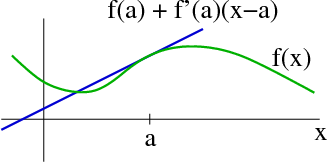# Math Insight

### Image: A tangent line to a graphA tangent to the graph of $f(x)$ at $x=0$.

Image file: tangent_line_graph.png